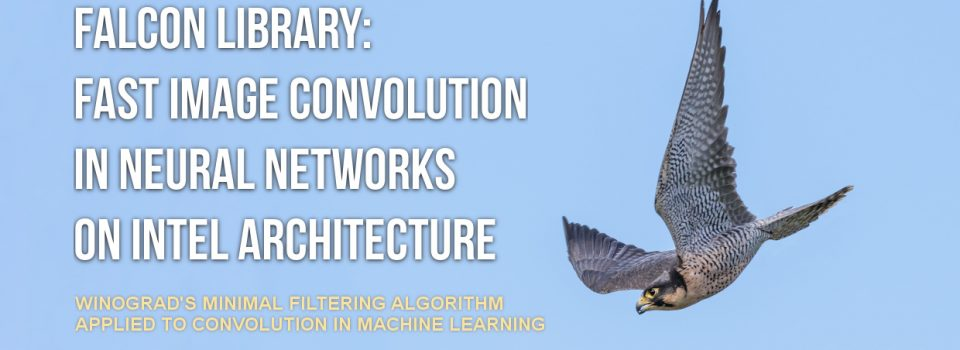We describe FALCON, an original open-source implementation of image convolution with a 3×3 filter based on Winograd’s minimal filtering algorithm. Compared to direct convolution, Winograd’s algorithm reduces the number of arithmetic operations at the cost of complicating the memory access pattern. This study is carried out in the context of image analysis in convolutional neural networks.

Our implementation combines C language code with BLAS function calls for general matrix-matrix multiplication. The code is optimized for Intel Xeon Phi processors x200 (formerly Knights Landing) with Intel Math Kernel Library (MKL) used for BLAS call to the SGEMM function.

To test the performance of FALCON in the context of machine learning, we benchmarked it for a set of image and filter sizes corresponding to the VGG Net architecture. In this test, FALCON achieves 10% greater overall performance than convolution from DNN primitives in Intel MKL. However, for some layers, FALCON is faster than MKL by 1.5x, but for other layers slower by as much as 4x. This indicates a possibility of a hybrid implementation with fast and direct convolution for a 30% speedup. High-bandwidth memory (MCDRAM) in Intel Xeon Phi x200 product family is a significant factor in the efficiency of the fast convolution algorithm.

Printable PDF:Colfax-Winograd-Summary.pdf (295 KB) — this file is available only to registered users. Register or Log In.
Code: github.com/ColfaxResearch/FALCON

Why are some sections grayed out? You are viewing an abridged version of this publication.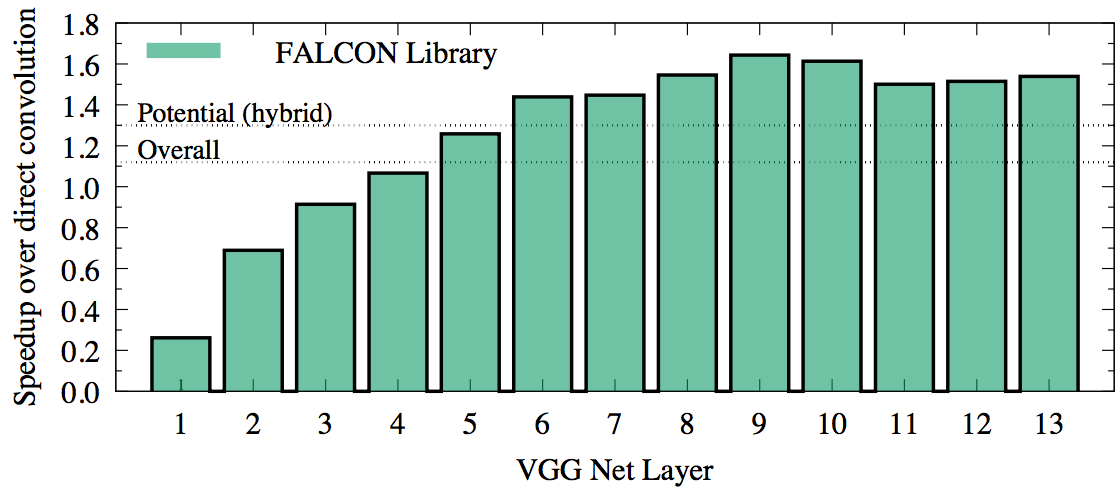## Section 1. Convolution in Machine Learning

Applications of deep neural networks (DNNs) to machine learning are diverse and promptly emerging, reaching the fields of assistive technologies, commerce, fundamental sciences, medical imaging and security (see, e.g., this article). DNNs thrive with abundant data. As a consequence, training DNNs often requires expensive development time and powerful computing resources. Therefore, even small improvements in the efficiency of the fundamental building blocks of DNNs can benefit the field of machine learning.

In image analysis with DNNs, one building block has gained particular importance in recent years: the operation of convolution of images with a filter. This operation is used in convolutional DNNs (ConvNets), which rely on the mathematical operation of convolution for position-independent object identification in images (see this article).

Numerically, convolution may be performed directly. This method is expensive in terms of computational complexity. For an image of size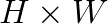$H \times W$ and filter of size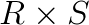$R \times S$, direct convolution requires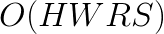$O(HWRS)$ operations. However, arithmetic operations in direct convolution can easily be collapsed to form the general matrix-matrix multiplication (GEMM) pattern (see this article). This simplifies the design of convolution functions because the complexity of memory and cache traffic management is delegated to the implementation of GEMM. Efficient GEMM code exists in Basic Linear Algebra Subroutine (BLAS) libraries for nearly every computer architecture. In the case of Intel architecture, Intel Math Kernel Library (MKL) has highly efficient implementation of GEMM and of direct convolution expressed with matrix-matrix multiplication.

At the same time, it is possible to compute convolution with alternative methods that perform fewer arithmetic operations than the direct method. For example, fast Fourier transform (FFT) may be used to compute image convolution with complexity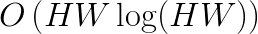$O\left(HW \log(HW) \right)$ (see this book). The asymptotic behavior of this algorithm predicts fewer operations than in direct method only if the filter is large enough: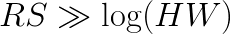$RS \gg \log (HW)$. However, this approach is not useful for ConvNets because they typically use filters as small as 2×2 or 3×3 pixels. In this range, the performance of the FFT method is poor compared to the direct method. In the domain of small filters, Winograd’s minimal filtering algorithm may be a better choice (see 1, 2). This approach has the same asymptotic complexity as the direct method,$O(HWRS)$, but it reduces the number of operations by a constant factor. In this paper we present the implementation of convolution based on Winograd’s minimal filtering algorithm for a filter size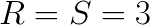$R=S=3$.

From here on, we refer to the convolution algorithm based on Winograd’s algorithm as “fast convolution”. This term, chosen by analogy with fast Fourier transform, signifies the algorithm performs fewer floating-point operations than the direct approach. At the same time, it is not trivial to implement a computer program performing “fast” convolution in less time than the direct method. This is because the fast algorithm requires data transformation with a complex memory access pattern, making it more difficult to express efficiently in code. The choice between an expensive yet simple algorithm (direct convolution) and less expensive but complicated algorithm (fast convolution) is not straightforward. It is difficult to predict, for instance, how well the hierarchy of memory and caches is able to serve the complex data access pattern of an algorithm based on strided or indirect memory access.

## Section 2. Winograd’s Minimal FIR Filtering

The original Winograd’s algorithm is applied to the computation of finite impulse response (FIR) filters. Direct application of 2 consecutive steps of a 3-tap FIR filter with coefficients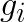$g_i$ to a set of 4 elements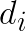$d_i$ requires 6 additions and 6 multiplications: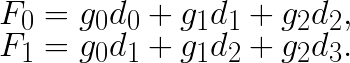$F_0 = g_0 d_0 + g_1 d_1 + g_2 d_2,\\F_1 = g_0 d_1 + g_1 d_2 + g_2 d_3.$

The idea of Winograd’s method is to compute these two filter outputs as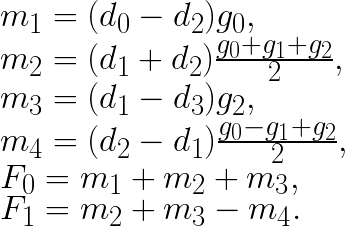$m_1 = (d_0 - d_2) g_0,\\ m_2 = (d_1 + d_2) \frac{g_0 + g_1 + g_2}{2},\\m_3 = (d_1 - d_3) g_2,\\m_4 = (d_2 - d_1) \frac{g_0 - g_1 + g_2}{2},\\F_0 = m_1 + m_2 + m_3,\\F_1 = m_2 + m_3 - m_4.$

If we precompute the expressions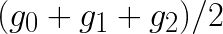$(g_0 + g_1 + g_2)/2$ and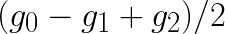$(g_0 - g_1 + g_2)/2$, then this procedure requires 8 additions and 4 multiplications, which is equal to number of floating point operations in the direct method. However, if our goal is to apply multiple filters gi to the same data di, then we can also precompute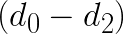$(d_0-d_2)$,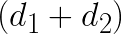$(d_1+d_2)$,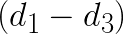$(d_1 - d_3)$ and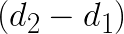$(d_2-d_1)$. With this done, the computation of F0 and F1 would only require 4 additions and 4 multiplications, yielding a speedup of (6+6)/(4+4)=1.5.

## Section 3. Application to ConvNets

In the context of ConvNets, the operation of convolution applies a total of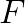$F$ filters of size$R \times S$ to a batch of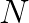$N$ images of size$H\times W$ with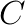$C$ channels in each. We enumerate filters with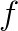$f$, and channels with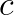$c$. Each image we split into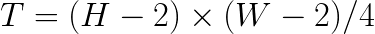$T=(H-2)\times (W-2)/4$ tiles and enumerate these tiles within the image with t, which ranges from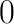$0$ to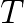$T$. The images within a batch are enumerated with n, which ranges from$0$ to$N$.

Direct convolution of a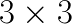$3\times 3$ filter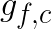$g_{f,c}$ with a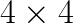$4 \times 4$ image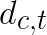$d_{c,t}$ to generate a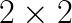$2 \times 2$ output tile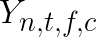$Y_{n,t,f,c}$ requires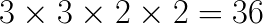$3 \times 3 \times 2 \times 2 = 36$ multiplications and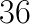$36$ additions. As shown by Lavin and Gray, Winograd’s fast FIR filter computation can be generalized to 2D filters, which are mathematically similar to convolution. The fast method can be expressed as shown below: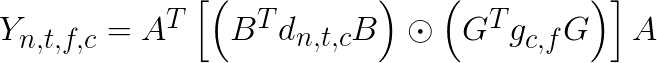$Y_{n,t,f,c} = A^{T}\left[ \left( B^{T}d_{n,t,c}B\right) \odot \left(G^{T}g_{c,f}G\right) \right]A$

where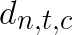$d_{n,t,c}$ is a$4 \times 4$ matrix representing the image tile,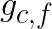$g_{c,f}$ is a$3 \times 3$ matrix representing channel$c$ of filter$f$,$Y_{n,t,f,c}$ is a$2\times 2$ matrix with the output of the convolution of$d_{n,t,c}$ with$g_{c,f}$,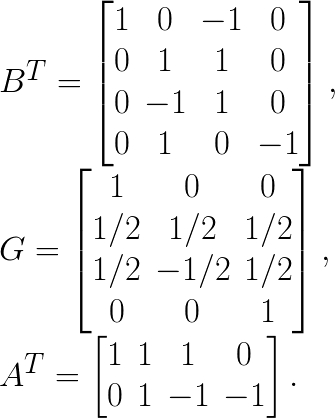$B^{T}=\begin{bmatrix} 1 & 0 & -1 & 0 \\ 0 & 1 & 1 & 0 \\ 0 & -1 & 1 & 0 \\ 0 & 1 & 0 & -1 \end{bmatrix},\\G= \begin{bmatrix} 1 & 0 & 0 \\ 1/2 & 1/2 & 1/2 \\ 1/2 & -1/2 & 1/2\\ 0 & 0 & 1 \end{bmatrix}, \\A^{T}= \begin{bmatrix} 1 & 1 & 1 & 0 \\ 0 & 1 & -1 & -1 \end{bmatrix}.$

In the above equation, the symbol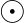$\odot$ indicates element-wise multiplication. Assuming that we have many image tiles and multiple filters, we can precompute transformed image tiles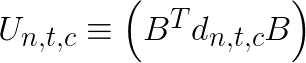$U_{n,t,c} \equiv \left(B^{T}d_{n,t,c} B\right)$ and transformed filters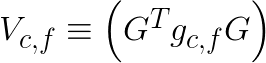$V_{c,f}\equiv\left(G^{T}g_{c,f}G\right)$. With that done, the algorithm requires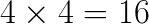$4\times4=16$ multiplications between the transformed input and filters. For inverse transformations, 24 additions are required. This is already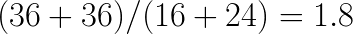$(36+36)/(16+24)=1.8$ times fewer operations than in the direct method. In addition to this, for the purposes of ConvNets, convolution must be applied to$C$ image channels, and results for individual channels must be summed: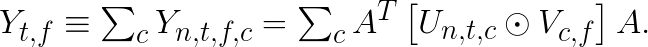$Y_{t,f} \equiv \sum_{c} Y_{n,t,f,c} = \sum_{c} A^T \left[ U_{n,t,c} \odot V_{c,f} \right] A.$

This allows one to perform the summation over channels first (16 additions per tile per channel) and apply inverse transformation after the summation,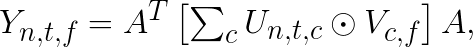$Y_{n,t,f} = A^T \left[ \sum_{c} U_{n,t,c} \odot V_{c,f} \right] A, \label{eq-sumfilters}$

thereby making its contribution to the operation count negligible. This results in net savings in the number of operations by a factor of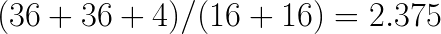$(36+36+4)/(16+16)=2.375$ (we factored in the need to do 4 additions per channel in the direct method).

The expectation of speedup in the fast algorithm due to reduced number of operations hinges on the assumption that the precomputation of the forward and backward transformation of data takes little time. In reality, our experiments revealed that the straightforward implementation of the above algorithm does not provide high performance, and platform-specific optimization is required.

## Section 4. Transformation to GEMM

In the above reasoning, we were making a silent assumption that the arithmetic throughput is the limiting factor of performance, i.e., memory traffic is completely overlapped with computation. This assumption holds true only if the order of tile convolutions is tuned to effectively re-use data in the processor’s caches. To avoid this complexity, as shown by Lavin and Gray, we we can express the arithmetic operations in the equations of the previous section as matrix multiplication, which allows us to delegate the complexity of overlapping memory traffic and computation to the GEMM function of a BLAS library.

Expressing the calculations through GEMM is possible because a transformed input tile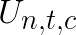$U_{n,t,c}$ can be reused to multiply with multiple corresponding filter tiles, and, similarly, a transformed filter tile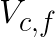$V_{c,f}$ can be reused to multiply with corresponding input tiles across all the batches. In addition, the input and filter tiles that are multiplied across$C$ channels are accumulated into one output tile. Denoting the elements of the$4 \times 4$ matrix$U_{n,t,c}$ as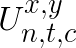$U^{x,y}_{n,t,c}$, and denoting elements of$V_{c,f}$ as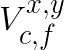$V^{x,y}_{c,f}$, we can define a$4\times 4$ matrix$P_{n,t,f}$ with elements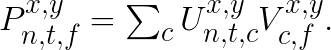$P^{x,y}_{n,t,f} = \sum_{c} U^{x,y}_{n,t,c} V^{x,y}_{c,f}.$

For each pair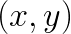$(x,y)$, this equation expresses the multiplication of matrix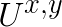$U^{x,y}$ by matrix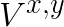$V^{x,y}$. The final result of convolution can then be written as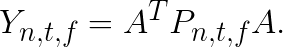$Y_{n,t,f} = A^T P_{n,t,f} A.$

To further improve performance, we collapse multiple matrices$U^{x,y}$, which results in the first matrix in GEMM having greater number of rows.

## Section 5. Implementation in Code

To compute convolution with the fast algorithm we follow approach similar to that shown by Lavin and Gray. There are three stages in this algorithm.

1. Input transformation: scattering on the image and filter data sets to form the input matrices.
2. Computation of product between transformed data and filter, and summation over channels expressed as GEMM, and
3. Output transformation: gathering the elements from the product matrices and their transformation to form the actual output of the convolution

Data transformation and the procedure for expressing the computation with GEMM are explained thoroughly by Lavin and Gray. We have modified the data layout and way the input matrices for GEMM are formed and the pseudo codes shown below illustrate the procedure.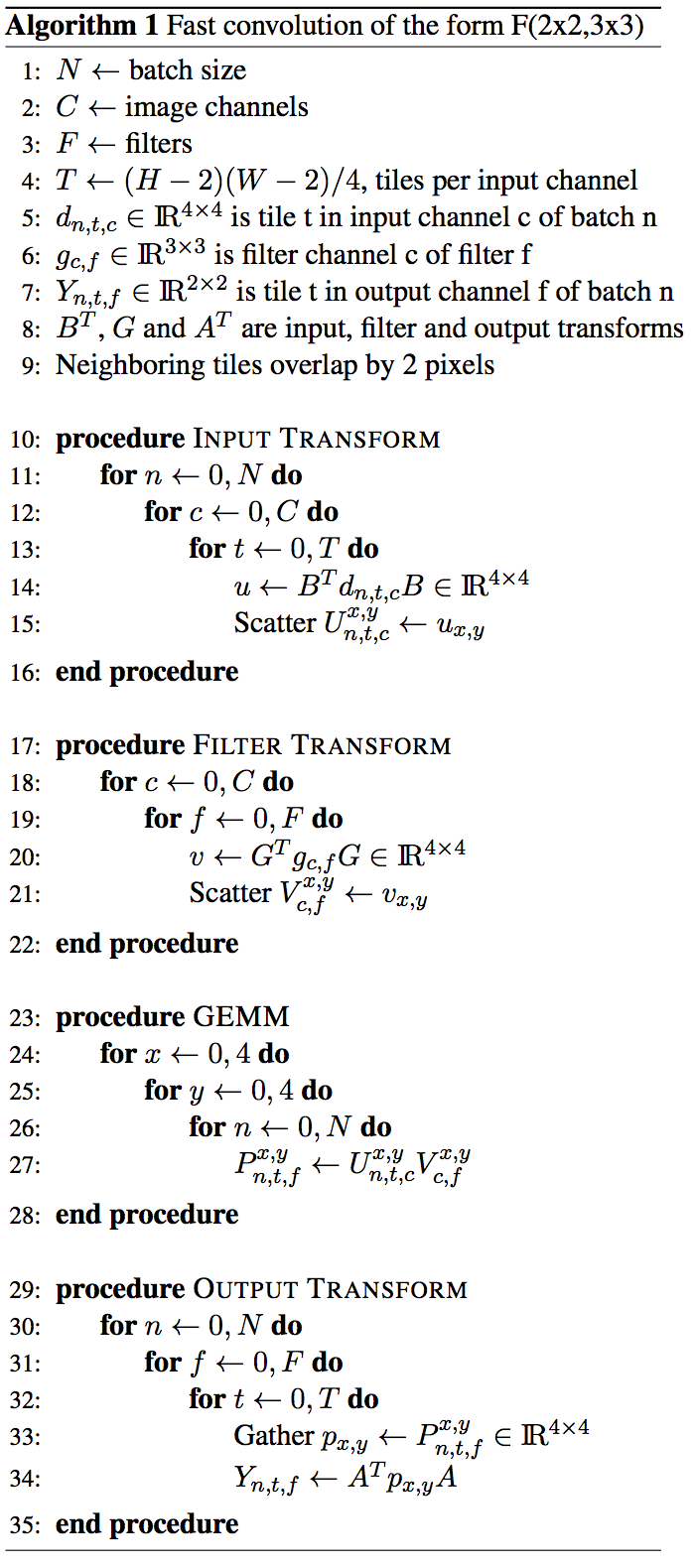The input data format is flexible and can be tuned with the help of merge factor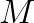$M$ to achieve high GEMM performance. Here,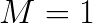$M=1$ results in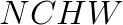$NCHW$ format,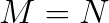$M=N$ results in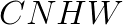$CNHW$ format, and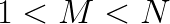$1 < M < N$ shuffles$N$ and$C$ keeping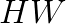$HW$ as fixed inner dimensions.

You are viewing an abridged version of this publication.